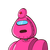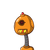# find how many litre of water will a cuboidal fish tank contain if its length breadth and height are 1 m , 40 cm and 50 cm​

find how many litre of water will a cuboidal fish tank contain if its length breadth and height are 1 m , 40 cm and 50 cm​

### 2 thoughts on “find how many litre of water will a cuboidal fish tank contain if its length breadth and height are 1 m , 40 cm and 50 cm​”

1.Step-by-step explanation:

Hi ,

Dimensions of the cuboidal fish tank :

length = l = 1 m = 100 cm

breadth = b = 40 cm

height = h = 50 cm

Volume of the tank ( V ) = lbh

V = 100 cm × 40cm × 50 cm

V = 200000 cm³

V = 200000 × 1/1000 litres

V = 200 litres

Therefore ,

Capacity of the water tank = 200 litres

I hope this helps you.

: )

2.• 200 litres

### Given:–

Dimensions of the cuboidal fish tank :

• length = l = 1 m = 100 cm
• breadth = b = 40 cm
• height = h = 50 cm

### Volume of the tank ( V ) = lbh

• => V = 100 cm × 40cm × 50 cm
• => V = 200000 cm³
• => V = 200000 × 1/1000 litres

### Therefore ,

Capacity of the water tank = 200 litres

I hope this helps you. !!